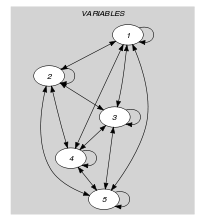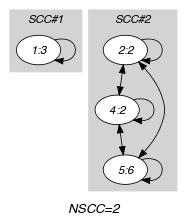## 5.271. nclass

Origin

Derived from $\mathrm{𝚗𝚟𝚊𝚕𝚞𝚎}$.

Constraint

$\mathrm{𝚗𝚌𝚕𝚊𝚜𝚜}\left(\mathrm{𝙽𝙲𝙻𝙰𝚂𝚂},\mathrm{𝚅𝙰𝚁𝙸𝙰𝙱𝙻𝙴𝚂},\mathrm{𝙿𝙰𝚁𝚃𝙸𝚃𝙸𝙾𝙽𝚂}\right)$

Type
 $\mathrm{𝚅𝙰𝙻𝚄𝙴𝚂}$ $\mathrm{𝚌𝚘𝚕𝚕𝚎𝚌𝚝𝚒𝚘𝚗}\left(\mathrm{𝚟𝚊𝚕}-\mathrm{𝚒𝚗𝚝}\right)$
Arguments
 $\mathrm{𝙽𝙲𝙻𝙰𝚂𝚂}$ $\mathrm{𝚍𝚟𝚊𝚛}$ $\mathrm{𝚅𝙰𝚁𝙸𝙰𝙱𝙻𝙴𝚂}$ $\mathrm{𝚌𝚘𝚕𝚕𝚎𝚌𝚝𝚒𝚘𝚗}\left(\mathrm{𝚟𝚊𝚛}-\mathrm{𝚍𝚟𝚊𝚛}\right)$ $\mathrm{𝙿𝙰𝚁𝚃𝙸𝚃𝙸𝙾𝙽𝚂}$ $\mathrm{𝚌𝚘𝚕𝚕𝚎𝚌𝚝𝚒𝚘𝚗}\left(𝚙-\mathrm{𝚅𝙰𝙻𝚄𝙴𝚂}\right)$
Restrictions
 $|\mathrm{𝚅𝙰𝙻𝚄𝙴𝚂}|\ge 1$ $\mathrm{𝚛𝚎𝚚𝚞𝚒𝚛𝚎𝚍}$$\left(\mathrm{𝚅𝙰𝙻𝚄𝙴𝚂},\mathrm{𝚟𝚊𝚕}\right)$ $\mathrm{𝚍𝚒𝚜𝚝𝚒𝚗𝚌𝚝}$$\left(\mathrm{𝚅𝙰𝙻𝚄𝙴𝚂},\mathrm{𝚟𝚊𝚕}\right)$ $\mathrm{𝙽𝙲𝙻𝙰𝚂𝚂}\ge 0$ $\mathrm{𝙽𝙲𝙻𝙰𝚂𝚂}\le \mathrm{𝚖𝚒𝚗}\left(|\mathrm{𝚅𝙰𝚁𝙸𝙰𝙱𝙻𝙴𝚂}|,|\mathrm{𝙿𝙰𝚁𝚃𝙸𝚃𝙸𝙾𝙽𝚂}|\right)$ $\mathrm{𝙽𝙲𝙻𝙰𝚂𝚂}\le$$\mathrm{𝚛𝚊𝚗𝚐𝚎}$$\left(\mathrm{𝚅𝙰𝚁𝙸𝙰𝙱𝙻𝙴𝚂}.\mathrm{𝚟𝚊𝚛}\right)$ $\mathrm{𝚛𝚎𝚚𝚞𝚒𝚛𝚎𝚍}$$\left(\mathrm{𝚅𝙰𝚁𝙸𝙰𝙱𝙻𝙴𝚂},\mathrm{𝚟𝚊𝚛}\right)$ $\mathrm{𝚛𝚎𝚚𝚞𝚒𝚛𝚎𝚍}$$\left(\mathrm{𝙿𝙰𝚁𝚃𝙸𝚃𝙸𝙾𝙽𝚂},𝚙\right)$ $|\mathrm{𝙿𝙰𝚁𝚃𝙸𝚃𝙸𝙾𝙽𝚂}|\ge 2$
Purpose

Number of partitions of the collection $\mathrm{𝙿𝙰𝚁𝚃𝙸𝚃𝙸𝙾𝙽𝚂}$ such that at least one value is assigned to at least one variable of the collection $\mathrm{𝚅𝙰𝚁𝙸𝙰𝙱𝙻𝙴𝚂}$.

Example
$\left(2,〈3,2,7,2,6〉,〈𝚙-〈1,3〉,𝚙-〈4〉,𝚙-〈2,6〉〉\right)$

Note that the values of $〈3,2,7,2,6〉$ occur within partitions $𝚙-〈1,3〉$ and $𝚙-〈2,6〉$ but not within $𝚙-〈4〉$. Consequently, the $\mathrm{𝚗𝚌𝚕𝚊𝚜𝚜}$ constraint holds since its first argument $\mathrm{𝙽𝙲𝙻𝙰𝚂𝚂}$ is set to value 2.

Typical
 $\mathrm{𝙽𝙲𝙻𝙰𝚂𝚂}>1$ $\mathrm{𝙽𝙲𝙻𝙰𝚂𝚂}<|\mathrm{𝚅𝙰𝚁𝙸𝙰𝙱𝙻𝙴𝚂}|$ $\mathrm{𝙽𝙲𝙻𝙰𝚂𝚂}<$$\mathrm{𝚛𝚊𝚗𝚐𝚎}$$\left(\mathrm{𝚅𝙰𝚁𝙸𝙰𝙱𝙻𝙴𝚂}.\mathrm{𝚟𝚊𝚛}\right)$ $|\mathrm{𝚅𝙰𝚁𝙸𝙰𝙱𝙻𝙴𝚂}|>|\mathrm{𝙿𝙰𝚁𝚃𝙸𝚃𝙸𝙾𝙽𝚂}|$
Symmetries
• Items of $\mathrm{𝚅𝙰𝚁𝙸𝙰𝙱𝙻𝙴𝚂}$ are permutable.

• Items of $\mathrm{𝙿𝙰𝚁𝚃𝙸𝚃𝙸𝙾𝙽𝚂}$ are permutable.

• Items of $\mathrm{𝙿𝙰𝚁𝚃𝙸𝚃𝙸𝙾𝙽𝚂}.𝚙$ are permutable.

• An occurrence of a value of $\mathrm{𝚅𝙰𝚁𝙸𝙰𝙱𝙻𝙴𝚂}.\mathrm{𝚟𝚊𝚛}$ can be replaced by any other value that also belongs to the same partition of $\mathrm{𝙿𝙰𝚁𝚃𝙸𝚃𝙸𝙾𝙽𝚂}$.

• All occurrences of two distinct tuples of values in $\mathrm{𝚅𝙰𝚁𝙸𝙰𝙱𝙻𝙴𝚂}.\mathrm{𝚟𝚊𝚛}$ or $\mathrm{𝙿𝙰𝚁𝚃𝙸𝚃𝙸𝙾𝙽𝚂}.𝚙.\mathrm{𝚟𝚊𝚕}$ can be swapped; all occurrences of a tuple of values in $\mathrm{𝚅𝙰𝚁𝙸𝙰𝙱𝙻𝙴𝚂}.\mathrm{𝚟𝚊𝚛}$ or $\mathrm{𝙿𝙰𝚁𝚃𝙸𝚃𝙸𝙾𝙽𝚂}.𝚙.\mathrm{𝚟𝚊𝚕}$ can be renamed to any unused tuple of values.

Arg. properties
• Functional dependency: $\mathrm{𝙽𝙲𝙻𝙰𝚂𝚂}$ determined by $\mathrm{𝚅𝙰𝚁𝙸𝙰𝙱𝙻𝙴𝚂}$ and $\mathrm{𝙿𝙰𝚁𝚃𝙸𝚃𝙸𝙾𝙽𝚂}$.

• Extensible wrt. $\mathrm{𝚅𝙰𝚁𝙸𝙰𝙱𝙻𝙴𝚂}$ when $\mathrm{𝙽𝙲𝙻𝙰𝚂𝚂}=|\mathrm{𝙿𝙰𝚁𝚃𝙸𝚃𝙸𝙾𝙽𝚂}|$.

Algorithm

related: $\mathrm{𝚗𝚎𝚚𝚞𝚒𝚟𝚊𝚕𝚎𝚗𝚌𝚎}$ ($\mathrm{𝚟𝚊𝚛𝚒𝚊𝚋𝚕𝚎}\in \mathrm{𝚙𝚊𝚛𝚝𝚒𝚝𝚒𝚘𝚗}$ replaced by $\mathrm{𝚟𝚊𝚛𝚒𝚊𝚋𝚕𝚎}\mathrm{mod}\mathrm{𝚌𝚘𝚗𝚜𝚝𝚊𝚗𝚝}$), $\mathrm{𝚗𝚒𝚗𝚝𝚎𝚛𝚟𝚊𝚕}$ ($\mathrm{𝚟𝚊𝚛𝚒𝚊𝚋𝚕𝚎}\in \mathrm{𝚙𝚊𝚛𝚝𝚒𝚝𝚒𝚘𝚗}$ replaced by $\mathrm{𝚟𝚊𝚛𝚒𝚊𝚋𝚕𝚎}/\mathrm{𝚌𝚘𝚗𝚜𝚝𝚊𝚗𝚝}$), $\mathrm{𝚗𝚙𝚊𝚒𝚛}$ ($\mathrm{𝚟𝚊𝚛𝚒𝚊𝚋𝚕𝚎}\in \mathrm{𝚙𝚊𝚛𝚝𝚒𝚝𝚒𝚘𝚗}$ replaced by $\mathrm{𝚙𝚊𝚒𝚛}$ of $\mathrm{𝚟𝚊𝚛𝚒𝚊𝚋𝚕𝚎𝚜}$).

specialisation: $\mathrm{𝚗𝚟𝚊𝚕𝚞𝚎}$ ($\mathrm{𝚟𝚊𝚛𝚒𝚊𝚋𝚕𝚎}\in \mathrm{𝚙𝚊𝚛𝚝𝚒𝚝𝚒𝚘𝚗}$ replaced by $\mathrm{𝚟𝚊𝚛𝚒𝚊𝚋𝚕𝚎}$).

Keywords
Arc input(s)

$\mathrm{𝚅𝙰𝚁𝙸𝙰𝙱𝙻𝙴𝚂}$

Arc generator
$\mathrm{𝐶𝐿𝐼𝑄𝑈𝐸}$$↦\mathrm{𝚌𝚘𝚕𝚕𝚎𝚌𝚝𝚒𝚘𝚗}\left(\mathrm{𝚟𝚊𝚛𝚒𝚊𝚋𝚕𝚎𝚜}\mathtt{1},\mathrm{𝚟𝚊𝚛𝚒𝚊𝚋𝚕𝚎𝚜}\mathtt{2}\right)$

Arc arity
Arc constraint(s)
$\mathrm{𝚒𝚗}_\mathrm{𝚜𝚊𝚖𝚎}_\mathrm{𝚙𝚊𝚛𝚝𝚒𝚝𝚒𝚘𝚗}$$\left(\mathrm{𝚟𝚊𝚛𝚒𝚊𝚋𝚕𝚎𝚜}\mathtt{1}.\mathrm{𝚟𝚊𝚛},\mathrm{𝚟𝚊𝚛𝚒𝚊𝚋𝚕𝚎𝚜}\mathtt{2}.\mathrm{𝚟𝚊𝚛},\mathrm{𝙿𝙰𝚁𝚃𝙸𝚃𝙸𝙾𝙽𝚂}\right)$
Graph property(ies)
$\mathrm{𝐍𝐒𝐂𝐂}$$=\mathrm{𝙽𝙲𝙻𝙰𝚂𝚂}$

Graph model

Parts (A) and (B) of Figure 5.271.1 respectively show the initial and final graph associated with the Example slot. Since we use the $\mathrm{𝐍𝐒𝐂𝐂}$ graph property we show the different strongly connected components of the final graph. Each strongly connected component corresponds to a class of values that was assigned to some variables of the $\mathrm{𝚅𝙰𝚁𝙸𝙰𝙱𝙻𝙴𝚂}$ collection. We effectively use two classes of values that respectively correspond to values $\left\{3\right\}$ and $\left\{2,6\right\}$. Note that we do not consider value 7 since it does not belong to the different classes of values we gave: all corresponding arc constraints do not hold.

##### Figure 5.271.1. Initial and final graph of the $\mathrm{𝚗𝚌𝚕𝚊𝚜𝚜}$ constraint(a) (b)